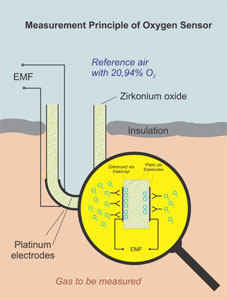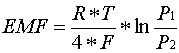## Measuring principle of oxygen

The oxygen measurement unit is designed to process signals from a stabilised zirconium oxide sensorZirconium oxide is a ceramic material, also characterised as a solid-state electrolyte, that has outstanding properties as an oxygen-ion conductor at high temperatures.

Within a certain temperature range, that depends on how the material is doped, such ionic conductors are able to fill the open spaces in their crystal grating with oxygen ions. The oxygen ions form on a conductive contact surface, generally of platinum, and thus the degree of oxygen activity is determined by the concentration of oxygen in the gas that is measured.In principle, the sensor is in the form of a solid-state electrolyte that is contacted on both sides, on the one side by a reference gas such as air and on the other by the gas to be measured. The sensor is so formed mechanically that the two gases are kept apart so that there is no possibility of them mixing.Calculation of the oxygen content (oxygen partial pressure) is carried out based on the measured voltage (emf) by transforming the following Nernst equation.Nernst equitation

Calculation is effected in accordance with the equation:whereby:

EMF = Electromotive force in Volts
R = 8.31J/mol K
T = Temperature in Kelvin
F = 96493 As/mol
P1 = Oxygen partial pressure on reference side at 0.20946 bar
P2 = Oxygen partial pressure on the measurement gas side

With above equation the beside diagram will result.